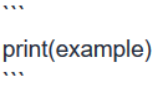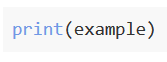# List and For Loops 10. The Average App Rating

``````opened_file = open('AppleStore.csv')

rating_sum = 0
for row in apps_data [1:]:
rating = float (row)
rating_sum = rating + rating_sum
avg_rating = rating_sum / len (row)
I have tried   avg_rating = rating_sum / len (rating)
len (row) and len (rating) are not working
the answer is avg_rating = rating_sum / len (apps_data[1:])
``````

Hello everyone,
I was wondering why I cannot use len (rating) or len (row) as denominator for the last line of code to calculate avg_rating? I think the numbers of these three lists are the same?

Thanks

The right answer is avg_rating = rating_sum / len (apps_data[1:])

I was wondering why avg_rating = rating_sum / len row)
or avg_rating = rating_sum / len len (rating)) were not working?Hey there deanwychange,

As a rule of thumb, instead of using screenshots it’s more helpful to copy-paste your code and use the appropriate formatting method to explain your question. In this case you’d envelop your code in 3 back ticks.

As an example:would appear asFrom the SS you posted, it looks like you tried to divide `rating_sum` by `len(row7)`. Try fixing that to `len(row)`.

1 Like

Both as advice to asking better questions and to improve your debugging skills, what exactly do you mean by “not working”? Does it mean the code does not run because of SyntaxError or something else? Most times, just running experiments/debugging print statements to clarify the information you need to ask a clearer question will solve the problem already.

was wondering why I cannot use len (rating) or len (row) as denominator for the last line of code to calculate avg_rating? I think the numbers of these three lists are the same?

Are the three options `len(rating)` , `len(row)` and the answer `len(apps_data[1:])` really the same? If you printed them out, what are their numbers? I expect the 1st two to be the same since `rating = float(row)` one is assigned from another, with just a float conversion. However, I also expected the 1st two to give you an error similiar to `TypeError: object of type 'int' has no len()`

Look at the output numbers and think about what the code is doing.(such as are you looking at things inside 1 iteration of a loop, or looking at the iterable object as a whole outside the loop?). Most objects in python are iterable, meaning you can run for loops through them and do something with it. Even for 2-dimensional objects like pandas dataframes, you can still iterate through, with each loop returning a column of information. This is to introduce the idea that not only single dimensional objects can be looped through. Put any object into `dir()` to see it’s attributes and methods which tells you what you can do with it. Use `type()` to see what type the object is and what happens if you iterate through them.

``````for element in iterable_object:
print(element)
``````

This is enough to get an idea of how any `iterable_object` is broken up if looped. Even if you don’t know if an object is iterable, doing it gives you the error message to confirm it is not.
Why do i keep saying for loops and iterables? Because len() is commonly used to find the length of iterable objects. Therefore, you could have used `len()` on any object too to check if it is iterable as a shortcut, and then use that code block above to go deeper to see what data structure is represented in each loop if it’s iterable.

Check exactly what is the type and value of the object you are passing into `len()` to make sure they produce the same as the answer. (sometimes having same value is sufficient to substitute code, but additionally, having same `type()` ensures you have the same methods available for use too, if required). This applies to anything else you debug, and to writing assert tests too in future.

Thanks so much.
It’s not just an answer but also let me know how to find the problem is, how to diagnose problem and how to understand python concepts more correctly!!

Please help mark as solved so others looking at the same problem in future does not have to scroll to find the solution: How do I mark a post as solved?

1 Like

opened_file = open(‘AppleStore.csv’)
rating_sum = 0
for each_row in apps_data[1:]:
rating = len(apps_data)
rating_sum = rating_sum + float(rating)

avg_rating = rating_sum / len(apps_data[1:])

I always get the value of agv is grater than expected I don’t get why my answer is so close from the answer

sum of float list >> 3537.2199999999875
So, it is not calculating correctly.
How do you sum it correctly? In previous exercises, it worked. But why not here?

opened_file = open(‘AppleStore.csv’)